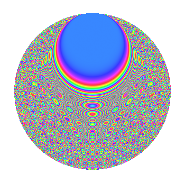# Properties

 Label 2100.2.dbLevel 2100 Weight 2 Character orbit db Rep. character $$\chi_{2100}(341,\cdot)$$ Character field $$\Q(\zeta_{30})$$ Dimension 640 Sturm bound 960

# Related objects

## Defining parameters

 Level: $$N$$ $$=$$ $$2100 = 2^{2} \cdot 3 \cdot 5^{2} \cdot 7$$ Weight: $$k$$ $$=$$ $$2$$ Character orbit: $$[\chi]$$ $$=$$ 2100.db (of order $$30$$ and degree $$8$$) Character conductor: $$\operatorname{cond}(\chi)$$ $$=$$ $$525$$ Character field: $$\Q(\zeta_{30})$$ Sturm bound: $$960$$

## Dimensions

The following table gives the dimensions of various subspaces of $$M_{2}(2100, [\chi])$$.

Total New Old
Modular forms 3936 640 3296
Cusp forms 3744 640 3104
Eisenstein series 192 0 192

## Trace form

 $$640q + 4q^{7} + O(q^{10})$$ $$640q + 4q^{7} - 26q^{15} - 6q^{21} - 2q^{25} + 18q^{31} + 45q^{33} + 8q^{37} + 2q^{39} - 32q^{43} + 87q^{45} + 20q^{49} - 12q^{51} - 124q^{57} + 25q^{63} + 16q^{67} - 24q^{73} + 93q^{75} + 28q^{79} - 4q^{81} - 92q^{85} + 18q^{87} - 2q^{91} + 22q^{93} + 32q^{99} + O(q^{100})$$

## Decomposition of $$S_{2}^{\mathrm{new}}(2100, [\chi])$$ into newform subspaces

The newforms in this space have not yet been added to the LMFDB.

## Decomposition of $$S_{2}^{\mathrm{old}}(2100, [\chi])$$ into lower level spaces

$$S_{2}^{\mathrm{old}}(2100, [\chi]) \cong$$ $$S_{2}^{\mathrm{new}}(525, [\chi])$$$$^{\oplus 3}$$$$\oplus$$$$S_{2}^{\mathrm{new}}(1050, [\chi])$$$$^{\oplus 2}$$

## Hecke characteristic polynomials

There are no characteristic polynomials of Hecke operators in the database